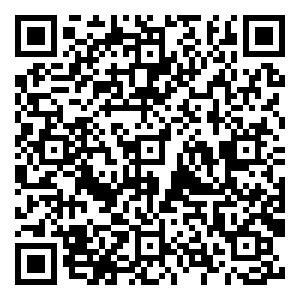• ISSN 2097-1893
• CN 10-1855/P

 引用本文: 陈阜斌，宗兆云，印兴耀. 2024. 基于固体声弹理论的地震反射透射研究进展. 地球与行星物理论评（中英文），55（0）：1-14 Chen F B, Zong Z Y, Yin X Y. 2024. Research progress on seismic reflection and transmission based on solid acoustoelasticity theory. Reviews of Geophysics and Planetary Physics, 55(0): 1-14 (in Chinese)## 基于固体声弹理论的地震反射透射研究进展

##### doi: 10.19975/j.dqyxx.2023-023

###### 通讯作者: 宗兆云（1987-），男，教授，主要从事油气储层地球物理的研究. E-mail：zongzhaoyun@upc.edu.cn
• 中图分类号: P315.0

## Research progress on seismic reflection and transmission based on solid acoustoelasticity theory

Funds: National Natural Science Foundation of China (Grant Nos. 42174139, 41974119, 42030103), the Laoshan Laboratory Science and Technology Innovation Program (Grant No. LSKJ202203406), the China Scholarship Council (Grant No. 202206450050), and the Innovation Fund Project for Graduate Students of China University of Petroleum (East China) (Grant No. 23CX04003A)
• 摘要: 多期沉积埋深及成岩过程使地下储层介质具有复杂初始地应力，应力范围跨度大且分布方向复杂. 初始地应力对储层介质岩石物理与地震波传播响应具有显著影响. 地震反射透射系数方程可以量化储层性质与地球物理观测数据的关系，构建考虑初始地应力作用的地震反射透射系数方程有利于更好理解深部储层界面处地震波传播特征，为深层油气勘探奠定理论基础，正逐渐受到国内外学者的关注. 当前已提出的相关理论模型主要从固体声弹理论出发，考虑初始应力与地震波扰动引起的微小固体应变，聚焦岩石物理与地震反射透射参数化的理论方法研究，在应力诱导各向异性参数反演、地应力预测、油气储层识别等方面开展了初步实际应用探索. 本文首先介绍固体声弹理论核心内容，然后介绍了基于声弹理论的地震反射透射模型、基本假设及其阶段性应用进展，讨论了方程的局限性以及在地震勘探领域的发展方向.

• 图  1  介质颗粒所处状态示意图. 图中${u^{\rm{i}}}$${u^{\rm{f}}}$$u$分别表示从自然状态到初始状态的质点位移、自然状态到最终状态的位移、初始状态到最终状态的位移. ${x_1}$${x_2}$${x_3}$为主坐标轴

Figure  1.  Sketch of the states of medium particle, where ${u^{\rm{i}}}$, ${u^{\rm{f}}}$and $u$represent particle displacements from natural state to initial state, natural state to final state, and initial state to final state, respectively. ${x_1}$, ${x_2}$and ${x_3}$are principal coordinate axes

图  2  实测干燥与饱和Berea砂岩$\rho {W^2}$的斜率（左图实心圆）与用右图所示3oECs预测的结果（左图空心圆）对比（修改自Winkler and McGowan, 2004）. 图中$\rho$$W$分别为密度和速度. PH和SH为静水压力下砂岩纵、横波速度，P2、S21和S32为单轴应力作用下纵、横波速度. A、B和C是三个独立的3oECs

Figure  2.  Comparison between the measured $\rho {W^2}$(solid circles in left panels) of Berea sandstone and the predicted results (open circles in left panels) with 3oECs shown in the right hand panels (modified from Winkler and McGowan, 2004), where $\rho$and $W$are the density and wave velocity of rock, respectively. PH and SH represent the P- and S-wave velocities in sandstone under hydrostatic pressure. P2, S21 and S32 represent one P-wave and two S-wave velocities in sandstone under the uniaxial stress. A, B and C are three independent 3oECs

图  3  单轴应力作用（a）与孔隙压力作用下（b）实测岩样速度（几何图形）与孔隙声弹模型预测结果（实线与虚线）对比图（修改自Liu et al., 2021）. 图中VP1为纵波速度，V31V32为两个不同方向的横波速度

Figure  3.  Comparisons between measured (geometries) and predicted (lines) velocities of rock sample under uniaxial stress (a) or pore pressure (b) (modified from Liu et al., 2021), where VP1 is P-wave velocity, V31 and V32 are S-wave velocities along two different directions.

图  4  岩石纵（a）、横（b）波速度随孔隙压力改变图（修改自Fu and Fu, 2018）. 图中虚线和实线分别为经典孔隙声弹理论和考虑软孔作用的新孔隙声弹理论预测的速度，空心圆为实测值

Figure  4.  P-wave (a) and S-wave (b) velocities of a rock sample variation with pore pressure (modified from Fu and Fu, 2018), where the dashed and solid lines represent the velocities provided by classic poro-acoustoelasticity theory and the new poro-acoustoelasticity theory with compliant pore, respectively. Circles represent the measured velocities

图  5  不同垂直应力T作用下连续界面纵波反射系数（a）、透射系数（b）与横波反射系数（c）、透射系数（d）随入射角变化图（修改自Chen et al., 2021

Figure  5.  P-wave reflection (a) and transmission (b) coefficients and S-wave reflection (c) and transmission (d) coefficients on the welded interface versus incidence angle under the different vertical in situ stresses (modified from Chen et al., 2021)

图  6  30°入射角时不同频率及垂直应力作用下非连续界面纵波反射系数（a）、透射系数（b）与横波反射系数（c）、透射系数（d）随法向界面柔度变化图（修改自Chen et al., 2022b）. 图中实线、虚线与点线分别表示20 Hz、40 Hz与60 Hz时的反射透射系数

Figure  6.  P-wave reflection (a) and transmission (b) coefficients and S-wave reflection (c) and transmission (d) coefficients on the nonwelded interface versus normal compliance under in situ stresses at different frequencies at an incidence angle of 30°(modified from Chen et al., 2022b), where the solid, dashed and dotted lines represent the reflection/transmission coefficients at frequencies of 20 Hz, 40 Hz and 60 Hz, respectively

图  7  随机噪声（a）与常噪声（b）条件下水平应力预测结果（修改自Chen et al., 2022c

Figure  7.  Prediction results of horizontal uniaxial stress under random noise (a) and constant noise (b) (modified from Chen et al., 2022c)

图  8  应力诱导各向异性因子反演结果（修改自Chen and Zong, 2022）. 其中图（a）、（b）分别为$100\times \left|\varPsi \right|$$100\times \left|Y\right|$反演结果. SIAF：应力诱导各向异性因子

Figure  8.  Inverted results of two stress-induced anisotropy factors (modified from Chen and Zong, 2022). (a) For $100\times \left|\varPsi \right|$and (b) for $100\times \left|Y\right|$图  9  应力诱导各向异性参数反演结果（修改自Pan and Zhao, 2023

Figure  9.  Inverted results of stress-induced anisotropy parameters (modified from Pan and Zhao, 2023)

•点击查看大图
##### 计量
• 文章访问数:  95
• HTML全文浏览量:  42
• PDF下载量:  36
• 被引次数: 0
##### 出版历程
• 收稿日期:  2023-05-08
• 修回日期:  2023-06-26
• 录用日期:  2023-06-26
• 网络出版日期:  2023-06-30

### 目录/下载:  全尺寸图片 幻灯片
• 分享
• 用微信扫码二维码

分享至好友和朋友圈